# Symmetric Function

Also found in: Wikipedia.

## symmetric function

[sə′me·trik ′fəŋk·shən]
(mathematics)
A function whose value is unchanged for any permutation of its variables.

## Symmetric Function

a function of two or more variables that remains unchanged under all permutations of the variables. Examples are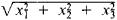and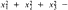4x1x2x3

Of particular importance in algebra are symmetric polynomials, especially elementary symmetric polynomials—that is, the functions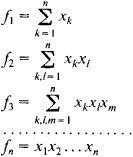where the summations are extended over all combinations of unequal numbers k, l, …. The sums are linear in each of the variables. According to the Vieta formulas, x1, x2,…,xn are the roots of the equation

xnf1 xn-1 + f2 xn-2 – … + (–1)nfn = 0

The fundamental theorem of the theory of symmetric polynomials states that any symmetric polynomial can be represented in one, and only one, way as a polynomial in the elementary symmetric polynomials: F (x1, x2,…. xn) = G (f1, f2,…, fn). If all the coefficients of Fare integers, then so are the coefficients of G. Thus, every symmetric polynomial with integer coefficients on the roots of an equation can be expressed as a polynomial with integer coefficients on the coefficients of that equation; for example.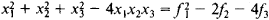Another important class of symmetric functions are the power sums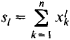The relation between such power sums and elementary symmetric polynomials is given by the Newton formulas: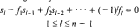and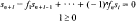These formulas permit the fk to be expressed successively in terms of the sm, and vice versa.

A function is said to be skew-symmetric, or alternating, if it remains unchanged under even permutations of x1, x2, …., xn and changes sign under odd permutations. Such functions can be rationally expressed in terms of f1, f2,…, fn and the product (seeDISCRIMINANT)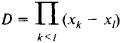the square of which is a symmetric function and thus can be rationally expressed in terms of f1, f2,…,fn

### REFERENCCE

Kurosh, A. G. Kurs vysshei algebry, 1 10th ed. Moscow, 1971.
Mentioned in ?
References in periodicals archive ?
Schur-Convex Functions and Inequalities; Volume 1: Concepts, Properties, and Applications in Symmetric Function Inequalities
For x = ([x.sub.1], ..., [x.sub.n]) [member of] [[0, 1).sup.n] = {x = ([x.sub.1], [x.sub.2], ..., [x.sub.n]) e [R.sup.n] : 0 [less than or equal to] [x.sub.j] < 1, i = 1, 2, ..., n} and r [member of] {1, 2, ..., n}, the symmetric function [F.sub.n](x, r) was defined by Guan  as
The homomorphism method derives generating functions for various permutation statistics by applying a ring homomorphism defined on the ring of symmetric functions [DELTA] in infinitely many variables [x.sub.1], [x.sub.2],[??] to simple symmetric function identities such as
If Q is a radially symmetric function such that [Q.sub.+] (0) = [Q.sub.+] ([infinity]) = 0, then problem ([P.sup.Q.sub.0]) has infinitely many solutions which are radially symmetric.
We refer to such functions in which all variables are symmetric as completely symmetric function. There are functions in which only some variables are symmetric giving rise to the concept of partially symmetric function.
Hotta-Springer  and Garsia-Procesi  discovered that the cohomology ring of the Springer fiber indexed by a partition p of n is isomorphic to certain quotient ring of F[X], which admits a graded [G.sub.n]-module structure corresponding to the modified Hall-Littlewood symmetric function [[??].sub.[mu]] (x; t) via the Frobenius characteristic map.
They define a symmetric function for each k-shape and show that they expand positively in terms of dual k-Schur functions, and obtain an explicit combinatorial description of the expansion of an ungraded k-Schur function into k+1 Schur functions.
Among the topics are the theory and application of plane partitions, linear homogeneous Diophantine equations and magic labelings of graphs, two combinatorial applications of the Aleksandrov-Fenchel inequalities, on the number of reduced decompositions of elements of Coxeter groups, and a symmetric function generalization of the chromatic polynomial of a graph.
We define the following symmetric function [F.sub.M,N]([z.sub.1], ...,[z.sub.N] [absolute value of [w.sub.1], ..., [w.sub.M]] [x.sub.1], ..., [x.sub.N]) which depends on the symmetric variables [z.sub.1], ..., [z.sub.N], complex parameters [w.sub.1], ...,w.sub.M], [[alpha].sub.1], ..., [[alpha].sub.M], [[gamma].sub.1], ..., [[gamma].sub.M] and integers [x.sub.1], ..., [x.sub.N] satisfying 1 [less than or equal to] [x.sub.1] < ...
is a symmetric function. Analogously, one can prove that any other term cosh[MATHEMATICAL EXPRESSION NOT REPRODUCIBLE IN ASCII] in Eq.
Adding one extra letter y to the argument of a symmetric function is a way to obtain a combinatorial description of this function.

Site: Follow: Share:
Open / Close